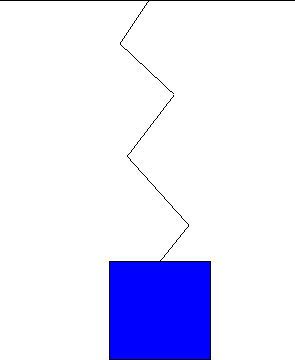Next: Electrical Potential Energy Up: Potential Energy Previous: Gravitational Potential Energy

## Elastic or Spring Potential Energy

Another type of conservative force'' is the force that a spring or an elastic exerts when stretched or pulled. Consider a mass connected to a spring which is then stretched a certain distance away from the spring's equilibrium point, as below.When the system is released the mass will oscillate up and down, and the spring will do work on the mass by virtue of the force that the spring exerts on the mass when it is stretched or compressed. This force is proportional to the distance the spring is stretched or compressed (at least for distances not too large), and the elastic potential energy associated with the force is proportional to the square of this distance.

In this situation we then have three types of energy - the kinetic energy of the mass, the potential energy of the spring, and the gravitational potential energy of the mass. The total energy is the sum of these three energies, and is conserved, but like before the individual forms of energy may transform amongst each other. For example, if pull the mass a certain distance down and release it from rest, initially the kinetic energy is zero. As it travels up, it gains speed and elevation, thus gaining kinetic and gravitational potential energy - this comes from a loss of the spring's elastic potential energy as the spring becomes less stretched. When the mass reaches the top, stops, and then starts coming back down, on the other hand, the gravitational potential energy gets transformed into kinetic energy and elastic potential energy of the stretching spring. At any given time, though, one finds the total energy - the sum of the kinetic, gravitational potential, and elastic potential - remains constant.Next: Electrical Potential Energy Up: Potential Energy Previous: Gravitational Potential Energy
modtech@theory.uwinnipeg.ca
1999-09-29